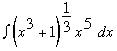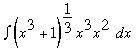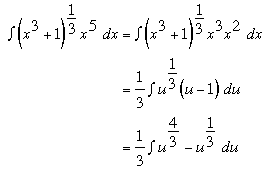SEARCH HOMEMath Central Quandaries & QueriesQuestion from Meghan, a student: Hi I have a question on indefinite integrals. I have been trying different u values, but I can't seem to cancel all the x's out. Evaluate the indefinite integral of (x^3 +1)^(1/3) * x^5 * dx. Thanks for your help! -MeghanHello Meghan,

The substitution for this integral requires a little trick to eliminate all of the x’s. You have the integralso doing your substitution you let u = x3 + 1 and then du = 3x2 dx and simplifying this you get 1/3 du = x2 dx. However in our integral we have an x5 not an x2, but we can split up the x’s in the integral a bit to make it easier for us. So we take our integral and split up the x’s=. Now we have an x2 from our du statement and we can get an x3 from our u statement. Since u = x3 + 1 then solving for x3 we get x3 = 1 - u now we can substitute. So our process looks like the following.Then from here you can integrate with respect to u, and then substitute u = x3 + 1 back in after you have integrated to reach your final solution.

I hope this helps. Happy integration.

Brennan YaremkoMath Central is supported by the University of Regina and The Pacific Institute for the Mathematical Sciences.